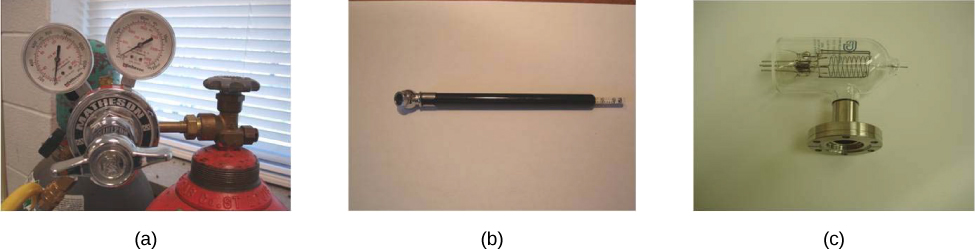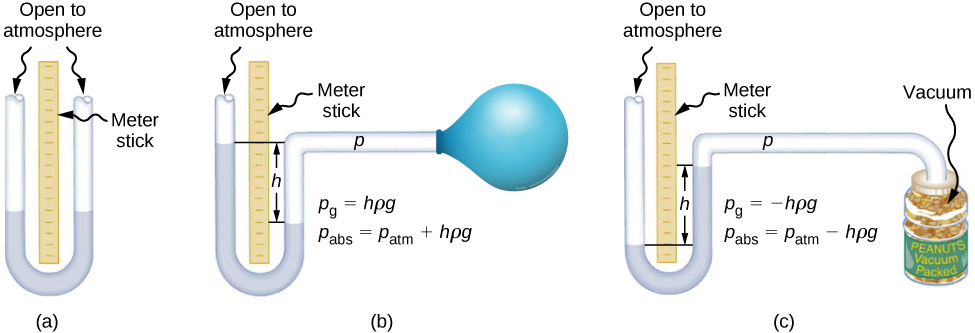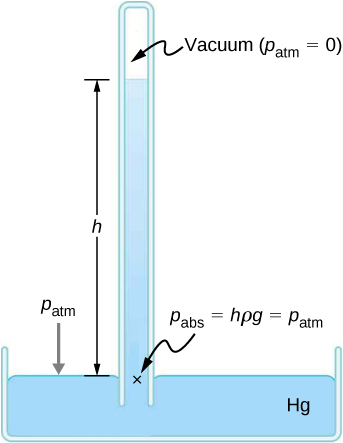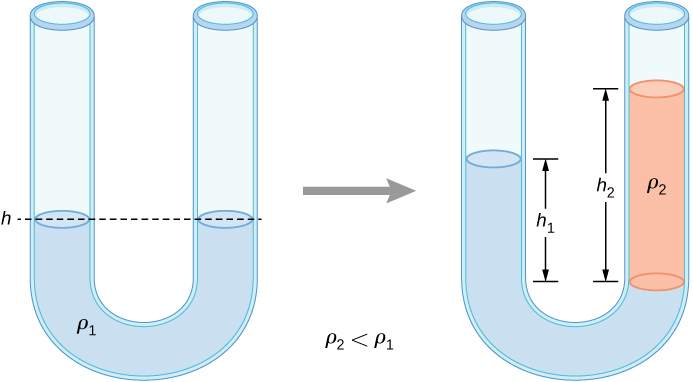Fluid Mechanics

# Measuring Pressure

### Learning Objectives

By the end of this section, you will be able to:

• Define gauge pressure and absolute pressure
• Explain various methods for measuring pressure
• Understand the working of open-tube barometers
• Describe in detail how manometers and barometers operate

In the preceding section, we derived a formula for calculating the variation in pressure for a fluid in hydrostatic equilibrium. As it turns out, this is a very useful calculation. Measurements of pressure are important in daily life as well as in science and engineering applications. In this section, we discuss different ways that pressure can be reported and measured.

### Gauge Pressure vs. Absolute Pressure

Suppose the pressure gauge on a full scuba tank reads 3000 psi, which is approximately 207 atmospheres. When the valve is opened, air begins to escape because the pressure inside the tank is greater than the atmospheric pressure outside the tank. Air continues to escape from the tank until the pressure inside the tank equals the pressure of the atmosphere outside the tank. At this point, the pressure gauge on the tank reads zero, even though the pressure inside the tank is actually 1 atmosphere—the same as the air pressure outside the tank.

Most pressure gauges, like the one on the scuba tank, are calibrated to read zero at atmospheric pressure. Pressure readings from such gauges are called gauge pressure, which is the pressure relative to the atmospheric pressure. When the pressure inside the tank is greater than atmospheric pressure, the gauge reports a positive value.

Some gauges are designed to measure negative pressure. For example, many physics experiments must take place in a vacuum chamber, a rigid chamber from which some of the air is pumped out. The pressure inside the vacuum chamber is less than atmospheric pressure, so the pressure gauge on the chamber reads a negative value.

Unlike gauge pressure, absolute pressure accounts for atmospheric pressure, which in effect adds to the pressure in any fluid not enclosed in a rigid container.

Absolute Pressure

The absolute pressure, or total pressure, is the sum of gauge pressure and atmospheric pressure:

${p}_{\text{abs}}={p}_{\text{g}}+{p}_{\text{atm}}$

where ${p}_{\text{abs}}$ is absolute pressure, ${p}_{\text{g}}$ is gauge pressure, and ${p}_{\text{atm}}$ is atmospheric pressure.

For example, if a tire gauge reads 34 psi, then the absolute pressure is 34 psi plus 14.7 psi (${p}_{\text{atm}}$ in psi), or 48.7 psi (equivalent to 336 kPa).

In most cases, the absolute pressure in fluids cannot be negative. Fluids push rather than pull, so the smallest absolute pressure in a fluid is zero (a negative absolute pressure is a pull). Thus, the smallest possible gauge pressure is ${p}_{\text{g}}=\text{−}{p}_{\text{atm}}$ (which makes ${p}_{\text{abs}}$ zero). There is no theoretical limit to how large a gauge pressure can be.

### Measuring Pressure

A host of devices are used for measuring pressure, ranging from tire gauges to blood pressure monitors. Many other types of pressure gauges are commonly used to test the pressure of fluids, such as mechanical pressure gauges. We will explore some of these in this section.

Any property that changes with pressure in a known way can be used to construct a pressure gauge. Some of the most common types include strain gauges, which use the change in the shape of a material with pressure; capacitance pressure gauges, which use the change in electric capacitance due to shape change with pressure; piezoelectric pressure gauges, which generate a voltage difference across a piezoelectric material under a pressure difference between the two sides; and ion gauges, which measure pressure by ionizing molecules in highly evacuated chambers. Different pressure gauges are useful in different pressure ranges and under different physical situations. Some examples are shown in (Figure).

(a) Gauges are used to measure and monitor pressure in gas cylinders. Compressed gases are used in many industrial as well as medical applications. (b) Tire pressure gauges come in many different models, but all are meant for the same purpose: to measure the internal pressure of the tire. This enables the driver to keep the tires inflated at optimal pressure for load weight and driving conditions. (c) An ionization gauge is a high-sensitivity device used to monitor the pressure of gases in an enclosed system. Neutral gas molecules are ionized by the release of electrons, and the current is translated into a pressure reading. Ionization gauges are commonly used in industrial applications that rely on vacuum systems.#### Manometers

One of the most important classes of pressure gauges applies the property that pressure due to the weight of a fluid of constant density is given by $p=h\rho g$. The U-shaped tube shown in (Figure) is an example of a manometer; in part (a), both sides of the tube are open to the atmosphere, allowing atmospheric pressure to push down on each side equally so that its effects cancel.

A manometer with only one side open to the atmosphere is an ideal device for measuring gauge pressures. The gauge pressure is ${p}_{\text{g}}=h\rho g$ and is found by measuring h. For example, suppose one side of the U-tube is connected to some source of pressure ${p}_{\text{abs}},$ such as the balloon in part (b) of the figure or the vacuum-packed peanut jar shown in part (c). Pressure is transmitted undiminished to the manometer, and the fluid levels are no longer equal. In part (b), ${p}_{\text{abs}}$ is greater than atmospheric pressure, whereas in part (c), ${p}_{\text{abs}}$ is less than atmospheric pressure. In both cases, ${p}_{\text{abs}}$ differs from atmospheric pressure by an amount $h\rho g,$ where $\rho$ is the density of the fluid in the manometer. In part (b), ${p}_{\text{abs}}$ can support a column of fluid of height h, so it must exert a pressure $h\rho g$ greater than atmospheric pressure (the gauge pressure ${p}_{\text{g}}$ is positive). In part (c), atmospheric pressure can support a column of fluid of height h, so ${p}_{\text{abs}}$ is less than atmospheric pressure by an amount $h\rho g$ (the gauge pressure ${p}_{\text{g}}$ is negative).

An open-tube manometer has one side open to the atmosphere. (a) Fluid depth must be the same on both sides, or the pressure each side exerts at the bottom will be unequal and liquid will flow from the deeper side. (b) A positive gauge pressure ${p}_{\text{g}}=h\rho g$ transmitted to one side of the manometer can support a column of fluid of height h. (c) Similarly, atmospheric pressure is greater than a negative gauge pressure ${p}_{\text{g}}$ by an amount $h\rho g$. The jar’s rigidity prevents atmospheric pressure from being transmitted to the peanuts.#### Barometers

Manometers typically use a U-shaped tube of a fluid (often mercury) to measure pressure. A barometer (see (Figure)) is a device that typically uses a single column of mercury to measure atmospheric pressure. The barometer, invented by the Italian mathematician and physicist Evangelista Torricelli (1608–1647) in 1643, is constructed from a glass tube closed at one end and filled with mercury. The tube is then inverted and placed in a pool of mercury. This device measures atmospheric pressure, rather than gauge pressure, because there is a nearly pure vacuum above the mercury in the tube. The height of the mercury is such that $h\rho g={p}_{\text{atm}}$. When atmospheric pressure varies, the mercury rises or falls.

Weather forecasters closely monitor changes in atmospheric pressure (often reported as barometric pressure), as rising mercury typically signals improving weather and falling mercury indicates deteriorating weather. The barometer can also be used as an altimeter, since average atmospheric pressure varies with altitude. Mercury barometers and manometers are so common that units of mm Hg are often quoted for atmospheric pressure and blood pressures.

A mercury barometer measures atmospheric pressure. The pressure due to the mercury’s weight, $h\rho g$, equals atmospheric pressure. The atmosphere is able to force mercury in the tube to a height h because the pressure above the mercury is zero.Fluid Heights in an Open U-Tube
A U-tube with both ends open is filled with a liquid of density ${\rho }_{1}$ to a height h on both sides ((Figure)). A liquid of density ${\rho }_{2}<{\rho }_{1}$ is poured into one side and Liquid 2 settles on top of Liquid 1. The heights on the two sides are different. The height to the top of Liquid 2 from the interface is ${h}_{2}$ and the height to the top of Liquid 1 from the level of the interface is ${h}_{1}$. Derive a formula for the height difference.

Two liquids of different densities are shown in a U-tube.Strategy
The pressure at points at the same height on the two sides of a U-tube must be the same as long as the two points are in the same liquid. Therefore, we consider two points at the same level in the two arms of the tube: One point is the interface on the side of the Liquid 2 and the other is a point in the arm with Liquid 1 that is at the same level as the interface in the other arm. The pressure at each point is due to atmospheric pressure plus the weight of the liquid above it.

$\begin{array}{c}\text{Pressure on the side with Liquid 1}={p}_{0}+{\rho }_{1}g{h}_{1}\hfill \\ \text{Pressure on the side with Liquid 2}={p}_{0}+{\rho }_{2}g{h}_{2}\hfill \end{array}$

Solution
Since the two points are in Liquid 1 and are at the same height, the pressure at the two points must be the same. Therefore, we have

${p}_{0}+{\rho }_{1}g{h}_{1}={p}_{0}+{\rho }_{2}g{h}_{2}.$

Hence,

${\rho }_{1}{h}_{1}={\rho }_{2}{h}_{2}.$

This means that the difference in heights on the two sides of the U-tube is

${h}_{2}-{h}_{1}=\left(1-\frac{{p}_{1}}{{p}_{2}}\right){h}_{2}.$

The result makes sense if we set ${p}_{2}={p}_{1},$ which gives ${h}_{2}={h}_{1}.$ If the two sides have the same density, they have the same height.

Check Your Understanding Mercury is a hazardous substance. Why do you suppose mercury is typically used in barometers instead of a safer fluid such as water?

The density of mercury is 13.6 times greater than the density of water. It takes approximately 76 cm (29.9 in.) of mercury to measure the pressure of the atmosphere, whereas it would take approximately 10 m (34 ft.) of water.

#### Units of pressure

As stated earlier, the SI unit for pressure is the pascal (Pa), where

$1\phantom{\rule{0.2em}{0ex}}\text{Pa}=1\phantom{\rule{0.2em}{0ex}}{\text{N/m}}^{2}.$

In addition to the pascal, many other units for pressure are in common use ((Figure)). In meteorology, atmospheric pressure is often described in the unit of millibars (mbar), where

$1000\phantom{\rule{0.2em}{0ex}}\text{mbar}=1\phantom{\rule{0.2em}{0ex}}×\phantom{\rule{0.2em}{0ex}}{10}^{5}\phantom{\rule{0.2em}{0ex}}\text{Pa}.$

The millibar is a convenient unit for meteorologists because the average atmospheric pressure at sea level on Earth is $1.013\phantom{\rule{0.2em}{0ex}}×\phantom{\rule{0.2em}{0ex}}{10}^{5}\phantom{\rule{0.2em}{0ex}}\text{Pa}=1013\phantom{\rule{0.2em}{0ex}}\text{mbar}=1\phantom{\rule{0.2em}{0ex}}\text{atm}$. Using the equations derived when considering pressure at a depth in a fluid, pressure can also be measured as millimeters or inches of mercury. The pressure at the bottom of a 760-mm column of mercury at $0\phantom{\rule{0.2em}{0ex}}\text{°C}$ in a container where the top part is evacuated is equal to the atmospheric pressure. Thus, 760 mm Hg is also used in place of 1 atmosphere of pressure. In vacuum physics labs, scientists often use another unit called the torr, named after Torricelli, who, as we have just seen, invented the mercury manometer for measuring pressure. One torr is equal to a pressure of 1 mm Hg.

Summary of the Units of Pressure
Unit Definition
SI unit: the Pascal $1\phantom{\rule{0.2em}{0ex}}\text{Pa}=1\phantom{\rule{0.2em}{0ex}}{\text{N/m}}^{2}$
English unit: pounds per square inch (${\text{lb/in.}}^{2}$ or psi) $1\phantom{\rule{0.2em}{0ex}}\text{psi}=6.895\phantom{\rule{0.2em}{0ex}}×\phantom{\rule{0.2em}{0ex}}{10}^{3}\phantom{\rule{0.2em}{0ex}}\text{Pa}$
Other units of pressure $\begin{array}{cc}\hfill 1\phantom{\rule{0.2em}{0ex}}\text{atm}& =760\phantom{\rule{0.2em}{0ex}}\text{mmHg}\hfill \\ & =1.013\phantom{\rule{0.2em}{0ex}}×\phantom{\rule{0.2em}{0ex}}{10}^{5}\phantom{\rule{0.2em}{0ex}}\text{Pa}\hfill \\ & =14.7\phantom{\rule{0.2em}{0ex}}\text{psi}\hfill \\ & =29.9\phantom{\rule{0.2em}{0ex}}\text{inches of Hg}\hfill \\ & =1013\phantom{\rule{0.2em}{0ex}}\text{mbar}\hfill \end{array}$
$1\phantom{\rule{0.2em}{0ex}}\text{bar}={10}^{5}\phantom{\rule{0.2em}{0ex}}\text{Pa}$
$1\phantom{\rule{0.2em}{0ex}}\text{torr}=1\phantom{\rule{0.2em}{0ex}}\text{mm Hg}=133.3\phantom{\rule{0.2em}{0ex}}\text{Pa}$

### Summary

• Gauge pressure is the pressure relative to atmospheric pressure.
• Absolute pressure is the sum of gauge pressure and atmospheric pressure.
• Open-tube manometers have U-shaped tubes and one end is always open. They are used to measure pressure. A mercury barometer is a device that measures atmospheric pressure.
• The SI unit of pressure is the pascal (Pa), but several other units are commonly used.

### Conceptual Questions

Explain why the fluid reaches equal levels on either side of a manometer if both sides are open to the atmosphere, even if the tubes are of different diameters.

The pressure of the atmosphere is due to the weight of the air above. The pressure, force per area, on the manometer will be the same at the same depth of the atmosphere.

### Problems

Find the gauge and absolute pressures in the balloon and peanut jar shown in (Figure), assuming the manometer connected to the balloon uses water and the manometer connected to the jar contains mercury. Express in units of centimeters of water for the balloon and millimeters of mercury for the jar, taking $h=0.0500\text{m}$ for each.

How tall must a water-filled manometer be to measure blood pressure as high as 300 mm Hg?

4.08 m

Assuming bicycle tires are perfectly flexible and support the weight of bicycle and rider by pressure alone, calculate the total area of the tires in contact with the ground if a bicycle and rider have a total mass of 80.0 kg, and the gauge pressure in the tires is $3.50\phantom{\rule{0.2em}{0ex}}×\phantom{\rule{0.2em}{0ex}}{10}^{5}\phantom{\rule{0.2em}{0ex}}\text{Pa}$.

### Glossary

absolute pressure
sum of gauge pressure and atmospheric pressure
gauge pressure
pressure relative to atmospheric pressure